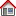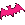Thread Zeitermittlung unter Perl? (10 answers) Opened by skontox at 2003-08-28 15:53 User since2003-08-08 2561 articles HausmeisterInHi! Code: (dl ) `1234567891011` `(\$sec, \$min, \$hours, \$day, \$month, \$year, \$wday, \$yday, \$isdst) = localtime();\$month += 1;\$year += 1900;\$min = "0\$min" if(\$min < "10");\$sec = "0\$sec" if(\$sec < "10");\$hours = "0\$hours" if(\$hours < "10");\$day = "0\$day" if(\$day < "10");\$month = "0\$month" if(\$month < "10");print "Heute ist der \$day.\$month.\$year. Es ist \$hours:\$min:\$sec Uhr!";` Gruß, Erik! s))91\&\/\^z->sub{}\(\@new\)=>69\&\/\^z->sub{}\(\@new\)=>124\&\/\^z->sub{}\(\@new\)=>); \$_.=qq~66\&\/\^z->sub{}\(\@new\)=>93~;for(@_=split(/\&\/\^z->sub{}\(\@new\)=>/)){print chr;} It's not a bug, it's a feature! - [CGI-World.de] View full thread Zeitermittlung unter Perl?